# Program to check Neon Number in Java

In this tutorial you will learn about the Program to check Neon Number in Java and its application with practical example.

In this tutorial, we will learn to create a Java Program to check Neon Numbers in Java using Java programming.

## Prerequisites

Before starting with this tutorial we assume that you are best aware of the following Java programming topics:

• Java Operators.
• Basic Input and Output function in Java.
• Class and Object in Java.
• Basic Java programming.
• If-else statements in Java.
• For loop in Java.

## What is Neon Number

A number is called a neon number if the sum of digits of the square of the number is equal to the number itself

For example:->

Square of “9 is 9*9 gives =>   81.
sum of digit of square : 8+1=9 (which gives the number it self).
So “9″ is a Neon Number.

## Program to check Neon Number in Java

In this program , we will find given number is a Neon number or not .First of all user will be prompted to enter Number and afterword we will find the given number is a Neon Number or not. Lets have look at the code.

## Output

Neon Number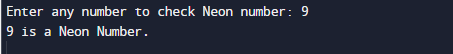Not a Neon Number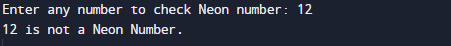In the above program, we have first declared and initialized a set variables required in the program.

• number = it will hold entered number.
• sqr =  it will calculate Square of a number.
• digit =  for finding digit of a number
• sum= sum of digit.

After that we take a number from user and find Neon number.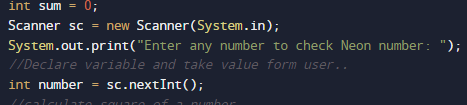after that we will find square of digit and sum of square digit value as shown in image below.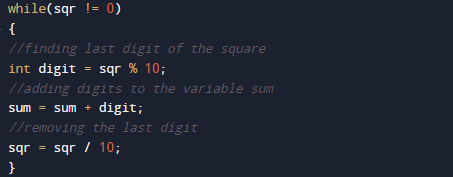then we check if sum of square digit equals to number then given number is Neon number.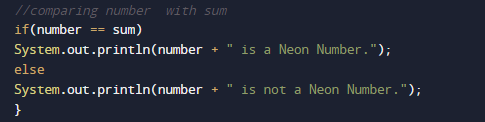In this tutorial we have learn about the Program to check Neon Number in Java and its application with practical example. I hope you will like this tutorial.